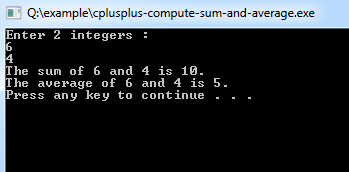## C++ Programming Examples Tutorial Index

This program takes in two integers x and y as a screen input from the user.

The sum and average of these two integers are calculated and outputted using the cout command.

Example:

``````#include <iostream>
using namespace std;

int main(){
int x,y,sum;
float average;

cout << "Enter 2 integers : " << endl;
cin>>x>>y;
sum=x+y;
average=sum/2;
cout << "The sum of " << x << " and " << y << " is " << sum << "." << endl;
cout << "The average of " << x << " and " << y << " is " << average << "." << endl;
}``````

Program Output:Explanation:

In this program, you will get to know about how within a program, you take two integer variables and find the sum and average of these two integer variables and display the output. So, first of all, you have to include the iostream header file using the "include" preceding by # which tells that hat the header file needs to be process before compilation, hence named preprocessor directive. And then, for removing naming conflict you can use namespace statement within a program.

Next is int main(). As you know that all the execution of any C++ program starts from main() function. So the main() function is defined with return type as integer. Now, you have to take three integer type variables name 'x', 'y' and 'sum'. Then you have to take another floating type variable name 'average'. Now using the cout statement prints the message "Enter 2 integers : " Next the cin statement takes the 2 values from the user and put them in x and y respectively. Then the addition of x and y is assigned to variable 'sum'. Also, the average variable is assigned with the value of sun divided by two (which is the formula for mean of two numbers).

Then the cout statements are use to display the calculated value of sum and average. The system("PAUSE") is used to pause the console of the system. And finally the return 0; statement is used to return an integer type value back to main().